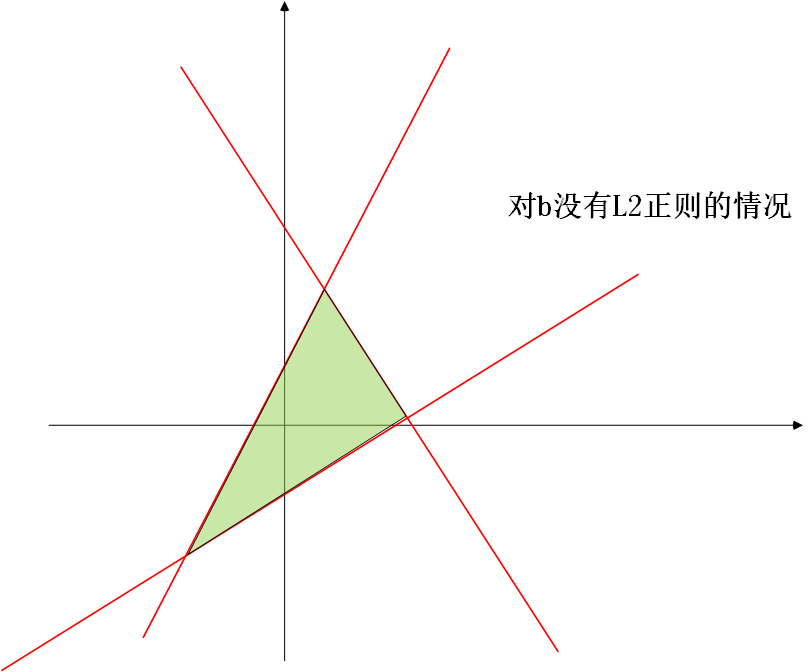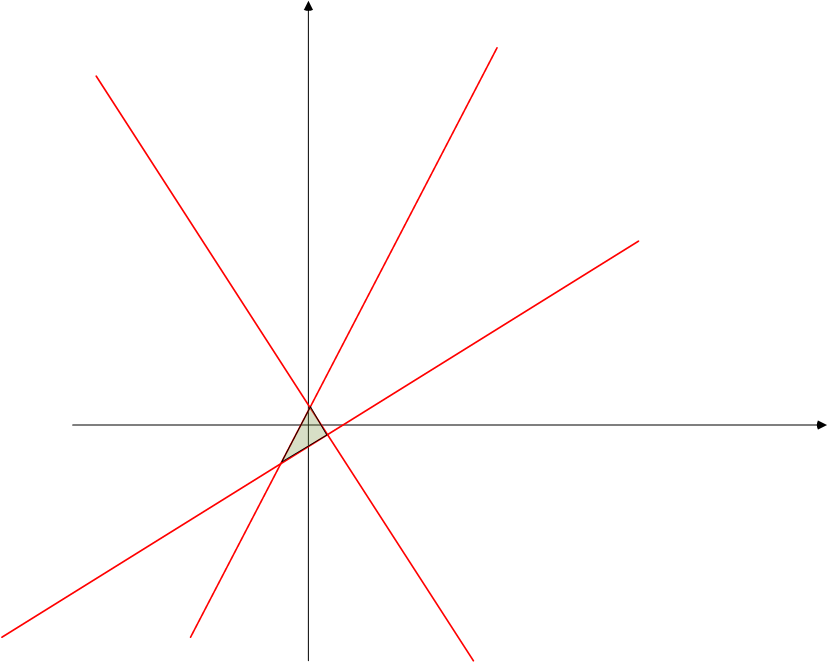# pytorch中的L2和L1正则化，自定义优化器设置等操作

∇ \nabla 联系方式：

e-mail: FesianXu@gmail.com

QQ: 973926198

github: https://github.com/FesianXuL r e g = ∣ ∣ y − y ^ ∣ ∣ 2 + λ ∣ ∣ W ∣ ∣ 2 (1) \mathcal{L}_{reg} = ||y-\hat{y}||^2+\lambda||W||^2 \tag{1}

torch.optim中包含了很多现成的优化器，包括SGD，Adadelta，Adam，Adagrad，RMSprop等，使用它很简单，你需要传入一个可迭代的参数列表（里面必须都是Variable类型的）进行优化，然后你可以指定一些优化器的参数，如学习率，动量，权值衰减等。例子如：

optimizer = optim.SGD(model.parameters(), lr = 0.01, momentum=0.9,weight_decay=1e-5)
optimizer = optim.Adam([var1, var2], lr = 0.0001)


optim.SGD([
{'params': model.base.parameters()},
{'params': model.classifier.parameters(), 'lr': 1e-3}
], lr=1e-2, momentum=0.9)


weight_p, bias_p = [],[]
for name, p in model.named_parameters():
if 'bias' in name:
bias_p += [p]
else:
weight_p += [p]
# 这里的model中每个参数的名字都是系统自动命名的，只要是权值都是带有weight，偏置都带有bias，
# 因此可以通过名字判断属性，这个和tensorflow不同，tensorflow是可以用户自己定义名字的，当然也会系统自己定义。
optim.SGD([
{'params': weight_p, 'weight_decay':1e-5},
{'params': bias_p, 'weight_decay':0}
], lr=1e-2, momentum=0.9)


Update 2018-12-12

import torch
import torch.nn as nn
import numpy as np

## build model
class net(nn.Module):
def __init__(self):
super().__init__()
self.fc1 = nn.Linear(100,50)
self.fc2 = nn.Linear(50,1)
self.relu = nn.ReLU(inplace=True)
def forward(self, inputs):
layer = self.fc1(inputs)
layer = self.relu(layer)
layer = self.fc2(layer)
return layer
## analoy inputs and labels
inputs = np.random.normal(size=(8,100))
inputs = torch.tensor(inputs).float()
labels = np.ones((8,1))
labels = torch.tensor(labels).float()

## update the weights and bias with L2 weight decay
n = net()
weight_p, bias_p = [],[]
for name, p in n.named_parameters():
if 'bias' in name:
bias_p += [p]
else:
weight_p += [p]

criterion = nn.MSELoss()
logit = n(inputs)
loss = criterion(input=logit, target=labels)
opt = torch.optim.SGD([{'params': weight_p, 'weight_decay':1e-5},
{'params': bias_p, 'weight_decay':0}],
lr=1e-2,
momentum=0.9)

## update
loss.backward()
opt.step()


Update 2019-9-30# Reference

1. Goodfellow I, Bengio Y, Courville A, et al. Deep learning[M]. Cambridge: MIT press, 2016. ↩︎

03-053453
07-2210
07-311万+
11-29484
04-291627
03-027337
04-163876
11-09114
08-225278
01-13460
03-221801
06-10335
08-15610
09-08853
12-171万+
01-29475
05-292210
05-03633
01-201156

### “相关推荐”对你有帮助么？

•非常没帮助
•没帮助
•一般
•有帮助
•非常有帮助被折叠的  条评论 为什么被折叠?到【灌水乐园】发言FesianXu

¥2 ¥4 ¥6 ¥10 ¥20余额支付 (余额：-- )扫码支付获取中扫码支付点击重新获取扫码支付1.余额是钱包充值的虚拟货币，按照1:1的比例进行支付金额的抵扣。
2.余额无法直接购买下载，可以购买VIP、C币套餐、付费专栏及课程。余额充值